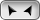## Welcome to OnlineConversion.com

Dynamic Viscosity Conversion

 Convert what quantity? From: centipoise decipoise dyne second/cm² gram-force second/cm² gram/centimeter second kilogram-force second/m²  kilogram/meter hour kilogram/meter second millipascal second millipoise newton second/m² pascal second poise poiseuille [France] pound-force second/ft² pound-force second/in² pound/foot hour pound/foot second poundal hour/ft² poundal second/ft² reyn slug/foot second To: centipoise decipoise dyne second/cm² gram-force second/cm² gram/centimeter second kilogram-force second/m²  kilogram/meter hour kilogram/meter second millipascal second millipoise newton second/m² pascal second poise poiseuille [France] pound-force second/ft² pound-force second/in² pound/foot hour pound/foot second poundal hour/ft² poundal second/ft² reyn slug/foot secondInformation:
The SI derived unit for dynamic viscosity is Pascal Second.
cm² - square centimeter
m² - square meter
ft² - square foot
in² - square inch

### BookMark Us

It may come in handy.

Are you bored?

Try the Fun Stuff